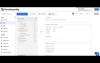# Form Calculations

### Introduction

Form fields can be computed using formulas. These formulas may reference other fields in your form using variables. Formulas are written in JavaScript and executed by the browser when filling out the form. These formulas and variables together are called Form Calculations

• For formulas to function properly, JavaScript must be enabled in the user's browser.
• Calculations can only be used in text fields.
• While FormAssembly does not have limits on the use of this feature, extensive use in combination with other, similar features may impact performance on respondent browsers and devices. Before you begin building advanced forms, we recommend reading through our Best Practices in Form Building document to learn more about our recommended planning and testing practices.

### Step 1: Define your Variables

A variable is a value that can be defined by an answer given in your form. A variable must be assigned to a question before it can be used in a formula. To define a variable, follow these steps:

1. Open a form in the Form Builder.
2. Click an existing question and use the Options button to open the editing sidebar.
3. Expand the Calculations tab. If you do not see a Calculations option, check the box to show advanced options.
4. Check the This is a variable box.
5. Enter a variable name for the question. Variable names may not contain spaces or non-alphabetic characters.  Please see the note below under "Create a Formula" for additional details on how to add a space if needed.
6. If you selected a multiple-choice question, you must provide a value for each choice.

### Step 2: Create a Formula

A formula is evaluated as the form is filled out, and the form is submitted with the computed value.

Form formulas are single-line JavaScript equations. They are mostly used to perform simple arithmetic, such as computing a total in an order form.

Please Note:  We are unable to provide support for custom JavaScript.

To create a computed field:

1. Open a form in the Form Builder.
2. Click an existing question and use the Options button to open the editing sidebar.
3. Expand the Calculations tab. If you do not see a Calculations option, check the box to show advanced options.
4. Check the box for This is a calculated field.
5. Enter an equation into the Formula text field.  You can use any variables you have defined from step 1 above.
Note: You can concatenate a string of variables by using "\u0020" to represent a space. For example, variableONE+“\u0020”+variableTWO+“\u0020”+variableTHREE

You can also add spaces to a variable value by adding \u0020 in the value. For example: "Variable\u0020One" Will be rendered as "Variable One"

### Video Tutorial### Examples

#### Simple formula performing arithmetic

`(a + b) / c * d`

#### 90% of the variable A, rounded to two decimals

`(A*0.9).toFixed(2)`

#### Calculating a Body Mass Index (BMI)

```height?((weight/(height*height))*703):0
// This example prevents a division by zero error by testing
// the height value first. It returns 0 if it's undefined or zero.```

#### Sample If/Else Calculation

`if(variableA=="Selection A"){variableA}else{variableB};`

#### Sample If/Else/Else If Calculation

`if(variableA=="Selection A"){variableA}else{if(variableA=="Selection B"){variableB}else{variableC}};`

### FAQ

##### I'm using Zip Codes in calculations, but the leading zero keeps getting stripped. How can I keep it at 5 digits?
`if(zip){('00000'+zip).substr(-5);}else{""};`